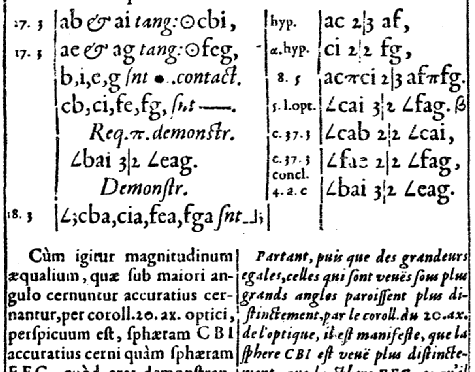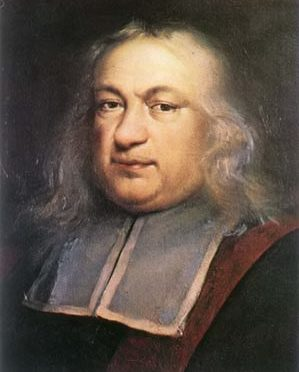# Tag Archives: pierre de fermat# Basque Fact of the Week: Pierre Hérigone, an Actual Basque Mathematician

Not long ago, I posted about mathematician Pierre de Fermat and his possible Basque connections (spoiler: he had none). However, in reading about this Pierre, I discovered another mathematician who certainly did have a Basque origin: Pierre Hérigone. His greatest contribution to math was in further developing the idea that math could be conveyed in […]# Basque Fact of the Week: Was the Mathematician Pierre de Fermat Basque?

Pierre de Fermat was one of the most recognized mathematicians of his time, and perhaps in all of history. He was infamous for making claims of mathematical proofs in the margins of documents without actually giving the proof. Ever since, mathematicians have struggled to prove his theorems. His Last Theorem, which relates to number theory, […]